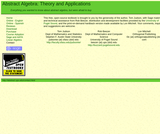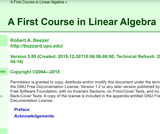# 2 Results

View
Selected filters:
• University of Puget SoundEducational Use
Rating

This text is intended for a one- or two-semester undergraduate course in abstract algebra. Traditionally, these courses have covered the theoretical aspects of groups, rings, and fields. However, with the development of computing in the last several decades, applications that involve abstract algebra and discrete mathematics have become increasingly important, and many science, engineering, and computer science students are now electing to minor in mathematics. Though theory still occupies a central role in the subject of abstract algebra and no student should go through such a course without a good notion of what a proof is, the importance of applications such as coding theory and cryptography has grown significantly.

Access also available here: http://abstract.ups.edu/contact.html

Preliminaries
The Integers
Groups
Cyclic Groups
Permutation Groups
Cosets and Lagrange's Theorem
Introduction to Cryptography
Algebraic Coding Theory
Isomorphisms
Normal Subgroups and Factor Groups
Homomorphisms
Matrix Groups and Symmetry
The Structure of Groups
Group Actions
The Sylow Theorems
Rings
Polynomials
Integral Domains
Lattices and Boolean Algebras
Vector Spaces
Fields
Finite Fields
Galois Theory

Subject:
Algebra
Material Type:
Textbook
Provider:
University of Puget Sound
Author:
Thomas Judson
01/01/2016Conditional Remix & Share Permitted
CC BY-SA
Rating

A First Course in Linear Algebra is an introductory textbook aimed at college-level sophomores and juniors. Typically students will have taken calculus, but it is not a prerequisite. The book begins with systems of linear equations, then covers matrix algebra, before taking up finite-dimensional vector spaces in full generality. The final chapter covers matrix representations of linear transformations, through diagonalization, change of basis and Jordan canonical form. Determinants and eigenvalues are covered along the way.

Systems of Linear Equations
Vectors
Matrices
Vector Spaces
Determinants
Eigenvalues
Linear Transformations
Representations
Preliminaries
Reference

Access also available here: http://linear.ups.edu/

Subject:
Mathematics
Algebra
Material Type:
Textbook
Provider:
University of Puget Sound
Author:
Robert Beezer